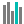#POISSON.DIST (PQL - xl)

This function returns the number of possible permutations for a specified number of items

• Library: PQL \ Spreadsheet \ Statistical
• Compatibility: Any content (regardless of data source) in the Tabulate spreadsheet module

#### Syntax

POISSON.DIST(x, mean, cumulative)

##### Function Arguments
 Name Description Type Optional x Number of events; provide an integer, or the value is truncated; the number must be greater than zero Number mean Expected numeric value; the number must be greater than zero Number cumulative Set to TRUE to return the cumulative Poisson probability that the number of random events occurring is between zero and nevents inclusive. Set to FALSE to return the Poisson probability mass function that the number of events occurring is exactly n events Number